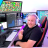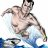# Remove cloud from MACD custom indicator

P

#### Parker427

##### New member
Can someone remove the "clouds" that are behind this indicator?

Code:
``````declare lower;

input fastLength = 12;
input slowLength = 26;
input MACDLength = 9;
input showBreakoutSignals = yes;
input ma_length = 21; #Length(180-200 for floating S/R , 55 for swing entry)

# Four Pole Filter
script g {
input length = 4;
input price = OHLC4;
def c;
def w;
def beta;
def alpha;
def G;
c = price;
w = (2 * Double.Pi / length);
beta = (1 - Cos(w)) / (Power(1.414, 2.0 / betaDev) - 1 );
alpha = (-beta + Sqrt(beta * beta + 2 * beta));
G = Power(alpha, 4) * c +
4 * (1 – alpha) * G – 6 * Power( 1 - alpha, 2 ) * G +
4 * Power( 1 - alpha, 3 ) * G - Power( 1 - alpha, 4 ) * G;
plot Line = G;
}
# Modified MACD
plot MACD_Value = g(length = fastLength) - g(length = slowLength);
MACD_Value.Hide();
plot MACD_Avg = g(price = MACD_Value, length = MACDLength);
MACD_Avg.Hide();

plot Diff = MACD_Value - MACD_Avg;
plot ZeroLine = 0;

MACD_Value.SetDefaultColor(GetColor(1));
MACD_Avg.SetDefaultColor(GetColor(8));
Diff.SetDefaultColor(GetColor(5));
Diff.SetPaintingStrategy(PaintingStrategy.HISTOGRAM);
Diff.SetLineWeight(3);
Diff.DefineColor("Positive and Up", Color.GREEN);
Diff.DefineColor("Positive and Down", Color.DARK_GREEN);
Diff.DefineColor("Negative and Down", Color.RED);
Diff.DefineColor("Negative and Up", Color.DARK_RED);
Diff.AssignValueColor(if Diff >= 0 then if Diff > Diff then Diff.Color("Positive and Up") else Diff.Color("Positive and Down") else if Diff < Diff then Diff.Color("Negative and Down") else Diff.Color("Negative and Up"));

ZeroLine.SetDefaultColor(GetColor(4));
ZeroLine.HideTitle();

plot UpSignal = if Diff crosses above ZeroLine then ZeroLine else Double.NaN;
plot DownSignal = if Diff crosses below ZeroLine then ZeroLine else Double.NaN;

UpSignal.SetHiding(!showBreakoutSignals);
Upsignal.SetLineWeight(3);
DownSignal.SetHiding(!showBreakoutSignals);
Downsignal.SetLineWeight(3);

UpSignal.SetDefaultColor(Color.UPTICK);
UpSignal.SetPaintingStrategy(PaintingStrategy.ARROW_UP);
UpSignal.HideTitle();
DownSignal.SetDefaultColor(Color.DOWNTICK);
DownSignal.SetPaintingStrategy(PaintingStrategy.ARROW_DOWN);
DownSignal.HideTitle();

# Forward / Reverse EMA
# (c) 2017 John F. Ehlers
# Ported to TOS 07.16.2017
# Mobius

# Inputs:
input AA = .1;

# Vars:
def CC;
def RE1;
def RE2;
def RE3;
def RE4;
def RE5;
def RE6;
def RE7;
def RE8;
def EMA;
plot EMA_Signal;
plot plot0;

CC = if CC == 0 then .9 else 1 – AA;
EMA = AA * close + CC * EMA;
RE1 = CC * EMA + EMA;
RE2 = Power(CC, 2) * RE1 + RE1;
RE3 = Power(CC, 4) * RE2 + RE2;
RE4 = Power(CC, 8) * RE3 + RE3;
RE5 = Power(CC, 16) * RE4 + RE4;
RE6 = Power(CC, 32) * RE5 + RE5;
RE7 = Power(CC, 64) * RE6 + RE6;
RE8 = Power(CC, 128) * RE7 + RE7;

EMA_Signal = EMA – AA * RE8;
EMA_Signal.AssignValueColor(if EMA_Signal > EMA_Signal
then Color.GREEN
else Color.RED);
EMA_Signal.SetLineWeight(3);

plot0 = if IsNaN(close) then Double.NaN else 0;
plot0.SetDefaultColor(Color.GRAY);
plot0.HideTitle();

# Multi-moving average Component

#input length = 14; #hint Length: Number of periods to average the data.
input movingAverageType = {default "Simple MA", "Exponential MA", "Wilders Smoothing", "Weighted MA", "Hull MA", "Adaptive MA", "Triangular MA", "Variable MA", "Dema MA", "Tema MA", "EHMA", "THMA"};
input displace = 0;

def avg_MACD_Value = ExpAverage(2 * ExpAverage(MACD_Value, ma_length / 2) - ExpAverage(MACD_Value, ma_length), Round(Sqrt(ma_length)));
def avg_MACD_Avg = ExpAverage(2 * ExpAverage(MACD_Avg, ma_length / 2) - ExpAverage(MACD_Avg, ma_length), Round(Sqrt(ma_length)));

plot X;
X.SetDefaultColor(CreateColor(0, 102, 204));
X.SetLineWeight(3);
plot Y;
Y.SetDefaultColor(Color.WHITE);
Y.SetLineWeight(3);

switch (movingAverageType) {
case "Simple MA":
X = Average(MACD_Value, ma_length);
Y = Average(MACD_Avg, ma_length);

case "Exponential MA":
X = ExpAverage(MACD_Value, ma_length);
Y = ExpAverage(MACD_Avg, ma_length);

case "Wilders Smoothing":
X = WildersAverage(MACD_Value, ma_length);
Y = WildersAverage(MACD_Avg, ma_length);

case "Weighted MA":
X = wma(MACD_Value, ma_length);
Y = wma(MACD_Avg, ma_length);

case "Hull MA":
X = HullMovingAvg(MACD_Value, ma_length);
Y = HullMovingAvg(MACD_Avg, ma_length);

case "Triangular MA":
X = MovAvgTriangular(MACD_Value, ma_length);
Y = MovAvgTriangular(MACD_Avg, ma_length);

case "Variable MA":
X = VariableMA(MACD_Value, ma_length);
Y = VariableMA(MACD_Avg, ma_length);

case "Dema MA":
X = DEMA(MACD_Value, ma_length);
Y = DEMA(MACD_Avg, ma_length);

case "Tema MA":
X = TEMA(MACD_Value, ma_length);
Y = TEMA(MACD_Avg, ma_length);

case EHMA:
X = ExpAverage(2 * ExpAverage(MACD_Value, ma_length / 2) - ExpAverage(MACD_Value, ma_length), Round(Sqrt(ma_length)));
Y = ExpAverage(2 * ExpAverage(MACD_Avg, ma_length / 2) - ExpAverage(MACD_Avg, ma_length), Round(Sqrt(ma_length)));

case THMA:
X = WMA(WMA(MACD_Value, (ma_length / 2) / 3) * 3 - WMA(MACD_Value, (ma_length / 2) / 2) - WMA(MACD_Value, (ma_length / 2)), (ma_length / 2));
Y = WMA(WMA(MACD_Avg, (ma_length / 2) / 3) * 3 - WMA(MACD_Avg, (ma_length / 2) / 2) - WMA(MACD_Avg, (ma_length / 2)), (ma_length / 2));
}
;``````

Last edited by a moderator:#### BenTen

Staff
VIP
Go to line 34 in the code and remove the following:

Code:
``addCloud(MACD_Value, MACD_Avg, Color.GREEN, Color.RED);``Remove Sub-Menu Bar from Charts Questions 2Moving average cloud? Questions 6Cloud above or below stock's opening price? Questions 2